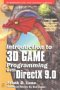# Maths - Exponent of Vectors

Here we calculate the exponent of a Vector. The result depends on whether the dimensions commute or anti-commute and whether the dimensions square to positive or negative. A summary of the results is given in the following table:

commutative square to result example derivation
anti-commute all positive exp(v) = cosh(|v|) + norm(v)*sinh(|v|) Euclidean vector see below
anti-commute all negative exp(v) = cos(|v|) + norm(v)*sin(|v|) bivector see below
commute two positive exp(x + Dy) = cosh(|v|) + D sinh(|v|) double number double number pages
commute one positive, one negative r e = r (cos(θ) + i sin(θ)) complex number complex number pages

where:

• |v| = √(v•v)) = magnitude scalar value
• norm(v) = v*(1/√(v•v)) = normalised (unit length) vector

To summarise and interpret the results, if the dimensions commute (as they do for complex numbers for example) then the result is a pure vector but, if the dimensions anti-commute (as they do for vectors in euclidean space for example) then the result is a scalar plus a vector. If this idea of adding scalars and vectors is a problem then see the pages about clifford (geometric) algebra, what this is saying is that the exponent does not have a solution within vector space but it does have a solution in clifford algebra.

in order to derive this result we will try two approaches:

However, before we start, it will be useful to review the formula for infinite series.

## Infinite Series

I have not found a version of Euler's equation which applies to vectors so we need to calculate it ourselves.

The only method that I can think of is to calculate the exponent using the series:

e(v) =
 ∞ ∑ n=0
 (v)n n!

Where:

• v = vector
• n= integer
• e = 2.71828

We have to be careful with vectors because they are not in general commutative. I think the above series applies but I'm not absolutely sure.

We now need to plug in a value for (v)n which we have calculated on this page.

 sin(x) x - x3/3! + x5/5! ... +(-1)rx2r+1/(2r+1)! all values of x cos(x) 1 - x2/2! + x4/4! ... +(-1)rx2r/(2r)! all values of x ln(1+x) x - x2/2! + x3/3! ... +(-1)r+1xr/(r)! -1 < x <= 1 exp(x) 1 + x1/1! + x2/2! + x3/3! ... + xr/(r)! all values of x exp(-x) 1 - x1/1! + x2/2! - x3/3! ... all values of x e 1 + 1/1! + 2/2! + 3/3! =2.718281828 sinh(x) x + x3/3! + x5/5! ... +x2r+1/(2r+1)! all values of x cosh(x) 1 + x2/2! + x4/4! ... +x2r/(2r)! all values of x

### Case 1: all dimensions square to positive

exp(v) = 1 + v1/1! + v2/2! + v3/3!+ v4/4! + v5/5! + …

So if all dimensions square to positive then:

v2 = v•v = positive scalar

so substituting gives:

exp(v) = 1 + v + v•v/2! + v•v*v/3!+ v•v*v•v/4! + v•v*v•v*v/5! + …

splitting up into real and vector parts gives:

exp(v) = 1 + v•v/2! + v•v*v•v/4! +…+ v*( 1+ v•v/3! + v•v*v•v/5! + …)

So we can see that it is a scalar + vector*another scalar

exp(v) = cosh(√(v•v)) + v*(1/√(v•v))*sinh(√(v•v))

### Case 2: all dimensions square to negative

exp(v) = 1 + v1/1! + v2/2! + v3/3!+ v4/4! + v5/5! + …

So if all dimensions square to negative then:

v2 = -v•v = negative scalar

so substituting gives:

exp(v) = 1 + v - v•v/2! - v•v*v/3!+ v•v*v•v/4! + v•v*v•v*v/5! + …

splitting up into real and vector parts gives:

exp(v) = 1 - v•v/2! + v•v*v•v/4! +…+ v*( 1- v•v/3! + v•v*v•v/5! + …)

So we can see that it is a scalar + vector*another scalar

exp(v) = cos(√(v•v)) + v*(1/√(v•v))*sin(√(v•v))

### Case 3: dimensions square to mixture of positive and negative

The difference between the above two cases depends on whether v2 = a positive or negative scalar.

So if we have a vector 'v' made up of the vectors x,y and z then to determine the nature of the exponent we calculate

s = v•v = x² + y ²+ z²

as follows:

√(v•v) exp(v)
if √(v•v) = +ve then: exp(v) = cosh(√(v•v)) + v/(√(v•v))*sinh(√(v•v))
if √(v•v) = -ve then: exp(v) = cos(√(v•v)) + v/(√(v•v))*sin(√(v•v))
if √(v•v) = 0 then: exp(v) = 1 + v

 metadata block see also: Correspondence about this page Book Shop - Further reading. Where I can, I have put links to Amazon for books that are relevant to the subject, click on the appropriate country flag to get more details of the book or to buy it from them.Introduction to 3D Game Programming with DirectX 9.0 - This is quite a small book but it has good concise information with subjects like, maths introduction and picking.If you are interested in 3D games, this looks like a good book to have on the shelf. If, like me, you want to have know the theory and how it is derived then there is a lot for you here. Including - Graphics pipeline, scenegraph, picking, collision detection, bezier curves, surfaces, key frame animation, level of detail, terrain, quadtrees & octtrees, special effects, numerical methods. Includes CDROM with code. This book contains more mathematically rigorous methods for picking than described above. Other Math Books Specific to this page here:

This site may have errors. Don't use for critical systems.